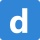Home
IT Knowledge
Inspiration
Languages
EN

# React - how to pass props to react components

3 points
Created by:Dirask Community
6240

In this article, we would like to show different ways how to pass props into React components.

Note: in the below example we use function components to show main idea.

Values can be passed as props using:

1. single or double apostrophes syntax (`''` or `""`) - that let us use only string values,
2. curly brackets syntax (`{}`) that let us pass:
• values with different types,
• variables,
3. spread syntax (`...`) that syntax that reduces the time needed to create a new component - read more about spread syntax here.

Quick solution:

``````const admin = { name: 'Tom', age: 30 };

<User name='Kate' age='25' />              // single apostrophes syntax
<User name="Kate" age="25" />              // double apostrophes syntax

<User name="John" age={25} />              // literals with curly brackets syntax
<User name="Chris" age={'25'} />           // literals with curly brackets syntax

Read below sections to see how different cases work in details.

## Practical examples

### 1. Single or double apostrophes example

JSX lets us use `''` or `""` when we pass props values. The main idea of this approach is to simplify string-valued props usage.

So component creation should look like:

``<User name="Kate" age="25" />``

Practical example:

``````// ONLINE-RUNNER:browser;

// Note: Uncomment import lines during working with JSX Compiler.
// import React from 'react';
// import ReactDOM from 'react-dom';

const User = ({ name, age }) => {
return (
<div>
<span>{name} ({typeof name})</span>,{' '}
<span>{age} ({typeof age})</span>
</div>
)
};

const App = () => {
return (
<div>
<User name="Kate" age="25" />
</div>
);
};

const root = document.querySelector('#root');
ReactDOM.render(<App />, root );``````

### 2. Curly brackets example

That approach lets us pass values with different types as props. Each prop can get value from literal or some variable.

So component creation should look like:

``````// const admin = { name: 'Tom', age: 30 };

<User name="John" age={25} />
<User name="Chris" age={'25'} />

Where `age={'25'}` is equal to `age="25"` syntax.

Practical example:

``````// ONLINE-RUNNER:browser;

// Note: Uncomment import lines during working with JSX Compiler.
// import React from 'react';
// import ReactDOM from 'react-dom';

const User = ({ name, age }) => {
return (
<div>
<span>{name} ({typeof name})</span>,{' '}
<span>{age} ({typeof age})</span>
</div>
)
};

const App = () => {
const admin = { name: 'Tom', age: 30 };
return (
<div className="App">
<User name="John" age={25} />
<User name="Chris" age={'25'} />
</div>
);
};

const root = document.querySelector('#root');
ReactDOM.render(<App />, root );``````

That case let us avoid typing many object props one after one and pass them using one operation.

So component creation should look like:

``````// const admin = { name: 'Tom', age: 30 };

Practical example:

``````// ONLINE-RUNNER:browser;

// Note: Uncomment import lines during working with JSX Compiler.
// import React from 'react';
// import ReactDOM from 'react-dom';

const User = ({ name, age }) => {
return (
<div>
<span>{name} ({typeof name})</span>,{' '}
<span>{age} ({typeof age})</span>
</div>
)
};

const App = () => {
const admin = { name: 'Tom', age: 30 };
return (
<div>
</div>
);
};

const root = document.querySelector('#root');
ReactDOM.render(<App />, root );``````